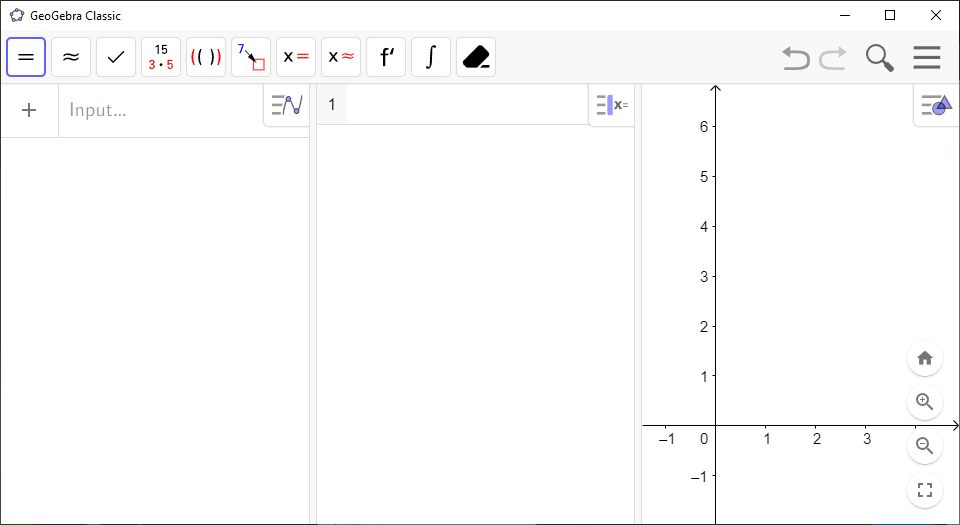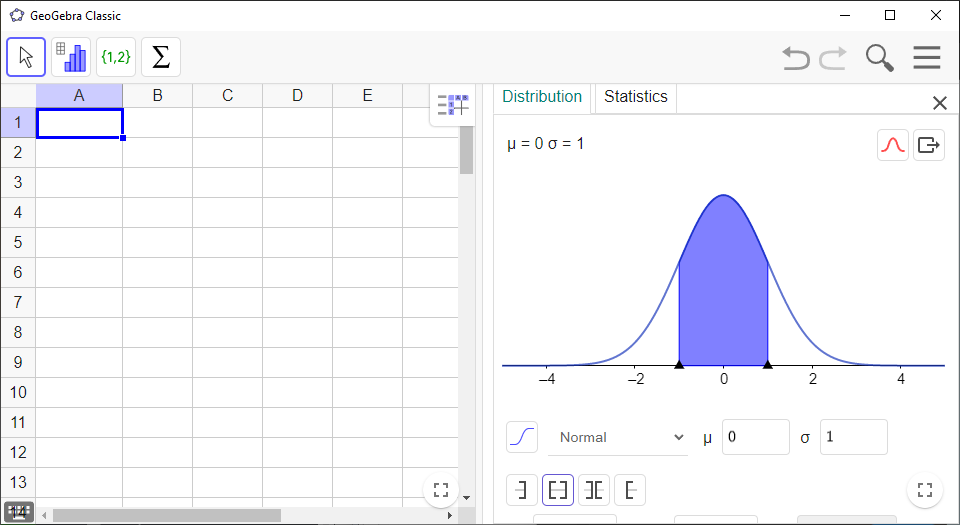Math Topics

# What Are GeoGebra Views?

When you open `GeoGebra`, you’ll see several windows. These are called views. If you don’t see any, you can find them under`View` in`Menu`. The most important ones are:

`Algebra View`

– Used to save expressions, functions and values. Objects that are in `CAS` are stored here as well.

`CAS` (Computer Algebra System)

– Used to perform calculator tasks, such as expanding algebraic expressions, solving equations, factorization, differentiation, integration and more.

`Graphics View`

– Used to plot graphs. You can easily change the name, style and layout of your graphs. Right-click the graph and click`Gear (Settings)`. Make any changes to your graphs here.`Spreadsheet`

– Used to make lists, perform regression analyses and conduct various statistical computations.

`Probability Calculator`– Used to compute probabilities.## Toolbars

All the views in `GeoGebra` have their own list of tools at the top, the `Toolbar` (F) for that specific view. If you click on any tool in the `Toolbar`, you’ll open a toolbox. The toolboxes are divided by category. For instance, the sixth toolbox in `Graphics View` contains tools for drawing circles.

The tools can do common tasks in mathematics for you. To use them, click on the icon within the boxes.

## Commands

`GeoGebra` also features tools in the form of commands. To execute a command, enter it in `CAS`. Some of the most common commands work in `Algebra View` as well. For example, if you want to factor the number 15, enter the command

Factor

in `CAS`, then select `Factor(<Number>)` from the list of suggestions. Replace the `<Number>` field with 15 and press `Enter`. `CAS` will print the answer $3\cdot 5$.

Many commands have multiple variations for different kinds of input. You probably saw that there are two variations of `Factor`: `Factor(<Number>)` and `Factor(<Polynomial>)`. If you insert a polynomial into `Factor(<Number>)`, the polynomial will actually not be factored! Make sure you pick the correct variation of the command.

## Saving Objects

Saving an object (like a function or a figure) in `GeoGebra` is known as defining that object.

You can define functions and variables to have specific expressions or variables in `GeoGebra` by typing

<Variable Name>=<Value or Expression>

in `Algebra View`, using one row for each object.

Example 1

To define the function $f\left(x\right)=2x+1$ in `Algebra View`, type `f=2x+1` in an empty row and press `Enter`.

Note! In `CAS`, you must type `:=` instead of `=` to define your objects. If you’re defining a function, you need to specify a name for its variable as well.

Example 2

To define the function $f\left(x\right)=2x+1$ in `CAS`, type `f(x):=2x+1` in an empty row and press `Enter`.